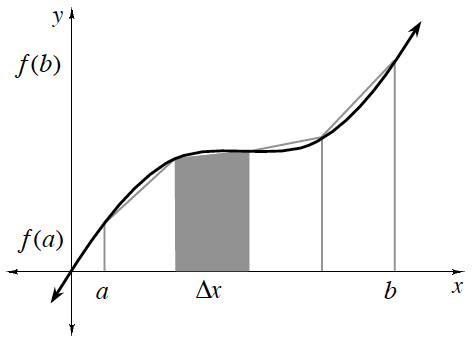### Home > APCALC > Chapter 3 > Lesson 3.3.3 > Problem3-121

3-121.

While testing the brakes of a new car, Badru recorded the following speeds traveled (in miles per hour) over time (in seconds). Approximately how far did Badru’s car travel before stopping?

 $\boldsymbol t$ (seconds) $0$ $1$ $2$ $3$ $4$ $5$ $6$ $7$ $8$ $9$ $10$ $\boldsymbol {v(t)}$ (mph) $50$ $48$ $46$ $43$ $40$ $37$ $34$ $29$ $23$ $14$ $0$

Notice the units. Time is given in seconds, but velocity is given in miles per hour.

Distance traveled can be found by calculating area under a velocity graph. Since we cannot see the graph and do not know the function, we must approximate the area given the select values in the table.

Which do you think will give the most accurate approximation: rectangles or trapezoids?

## The Trapezoid Rule

The Trapezoid Rule can be used to approximate the area under a curve if the widths, $\Delta x$, of all trapezoids are of equal size:

$\left. \begin{array} { r } { A = \frac { \Delta x } { 2 } ( f ( a ) + 2 f ( a + \Delta x ) + 2 f ( a + 2 \Delta x ) + \ldots } \\ { + 2 f ( b - \Delta x ) + f ( b ) ) } \end{array} \right.$